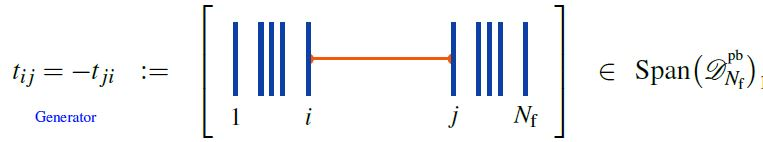Contents

# Contents

## In quantum mechanics

In quantum mechanics what is called the Dyson formula is what in mathematics is called the iterated integral-expression for parallel transport: It an expression for the solution to a differential equation of the form

$\frac{d}{d t} \psi(t) \;=\; i H(t) \psi(t)$

where $t \mapsto \psi(t) \in \mathcal{H}$ is a one-parameter family of elements of some Hilbert space and $t \mapsto H(t)$ is a one-parameter family of linear operators on this Hilbert space.

This appears prominently in the expression of the Schrödinger equation in the interaction picture, in which case $H(t)$ is the interaction Hamiltonian in the Heisenberg picture of the free theory. In this case the Dyson series gives the S-matrix. This is the context in which the term “Dyson formula” orginates. But of course also the plain Schrödinger equation (“in the Schrödinger picture”) is already of this form if the Hamiltonian is time-dependent.

The idea is to think of the solution as given by the limit

$\psi(t) \;=\; \underset{N \to \infty}{\lim} \underset{N \, \text{factors}}{ \underbrace{ \left( id + \tfrac{i}{N}H(t) \right) \left( id + \tfrac{i}{N}H(t(N-1)/N) \right) \cdots \left( id + \tfrac{i}{N}H(t/N) \right) } } \psi(0)$

and to think of this as the result of forming the exponential expression $\exp(\int_{[0,t] } H(t)\,d t)$ and then re-ordering in the resulting sum of products all the factors of $H(t)$ such that they are time ordered with larger values of $t$ ordered to the left of smaller values.

Accordingly, in the physics literature solutions to this equation are written

$\psi(t) \;=\; T\left( \exp\left( \int_{[0,t]} i H(t) \,d t \right) \right) \psi(0) \,,$

with $T(-)$ the time ordering operator producing time-ordered products. The corresponding series of iterated integrals

$\psi(t) \;=\; \left( \underoverset{n = 0}{\infty}{\sum} (i)^n \underset{0 \leq t_1 \leq \cdots \leq t_n \leq t}{\int} H(t_n) H(t_{n-1})\cdots H(t_1) \right)\psi(0)$

is called the Dyson series.

## In quantum field theory

The idea generalizes to relativistic field theory perturbative quantum field theory on Lorentzian spacetimes if due care is exercised (including adiabatic switching and point extension of operator-valued distributions). Here the “time ordering” is generalized to a causal ordering, this is the content of the construction of the S-matrix and the interacting field algebra in causal perturbation theory.

See at time-ordered product and S-matrix for details.

## Examples

### Kontsevich integral is Dyson formula of KZ-connection

For the Definition of the Knizhnik-Zamolodchikov connection we need the following notation:

1. configuration spaces of points

For $N_{\mathrm{f}} \in \mathbb{N}$ write

(1)$\underset{{}^{\{1,\cdots,N_{\mathrm{f}}\}}}{Conf}(\mathbb{R}^2) \;\coloneqq\; (\mathbb{R}^2)^n \backslash FatDiagonal$

for the ordered configuration space of n points in the plane, regarded as a smooth manifold.

Identifying the plane with the complex plane $\mathbb{C}$, we have canonical holomorphic coordinate functions

(2)$(z_1, \cdots, z_{N_{\mathrm{f}}}) \;\colon\; \underset{{}^{\{1,\cdots,n\}}}{Conf}(\mathbb{R}^2) \longrightarrow \mathbb{C}^{N_{\mathrm{f}}} \,.$
2. horizontal chord diagrams

(3)$\mathcal{A}^{{}^{pb}}_{N_{\mathrm{f}}} \;\coloneqq\; Span\big(\mathcal{D}^{{}^{pb}}_{N_{\mathrm{f}}}\big)/4T$

for the quotient vector space of the linear span of horizontal chord diagrams on $n$ strands by the 4T relations (infinitesimal braid relations), regarded as an associative algebra under concatenation of strands (here).

###### Definition

(Knizhnik-Zamolodchikov form)

(4)$\omega_{KZ} \;\in\; \Omega \big( \underset{{}^{\{1,\cdots,N_{\mathrm{f}}\}}}{Conf}(\mathbb{C}) \,, \mathcal{A}^{{}^{pb}}_{N_{\mathrm{f}}} \big)$

given in the canonical coordinates (2) by:

(5)$\omega_{KZ} \;\coloneqq\; \underset{ i \lt j \in \{1, \cdots, n\} }{\sum} d_{dR} log\big( z_i - z_j \big) \otimes t_{i j} \,,$

whereis the horizontal chord diagram with exactly one chord, which stretches between the $i$th and the $j$th strand.

Regarded as a connection form for a connection on a vector bundle, this defines the universal Knizhnik-Zamolodchikov connection $\nabla_{KZ}$, with covariant derivative

$\nabla \phi \;\coloneqq\; d \phi + \omega_{KV} \wedge \phi$

for any smooth function

$\phi \;\colon\; \underset{{}^{\{1,\cdots,N_{\mathrm{f}}\}}}{Conf}(\mathbb{C}) \longrightarrow \mathcal{A}^{{}^{pb}}_{N_{\mathrm{f}}} Mod$

with values in modules over the algebra of horizontal chord diagrams modulo 4T relations.

The condition of covariant constancy

$\nabla_{KZ} \phi \;=\; 0$

is called the Knizhnik-Zamolodchikov equation.

Finally, given a metric Lie algebra $\mathfrak{g}$ and a tuple of Lie algebra representations

$( V_1, \cdots, V_{N_{\mathrm{f}}} ) \;\in\; (\mathfrak{g} Rep_{/\sim})^{N_{\mathrm{f}}} \,,$

the corresponding endomorphism-valued Lie algebra weight system

$w_{V} \;\colon\; \mathcal{A}^{{}^{pf}}_{N_{\mathrm{f}}} \longrightarrow End_{\mathfrak{g}}\big( V_1 \otimes \cdots V_{N_{\mathrm{f}}} \big)$

turns the universal Knizhnik-Zamolodchikov form (4) into a endomorphism ring-valued differential form

(6)$\omega_{KZ} \;\coloneqq\; \underset{ i \lt j \in \{1, \cdots, n\} }{\sum} d_{dR} log\big( z_i - z_j \big) \otimes w_V(t_{i j}) \;\in\; \Omega \big( \underset{{}^{\{1,\cdots,N_{\mathrm{f}}\}}}{Conf}(\mathbb{C}) \,, End\big(V_1 \otimes \cdot V_{N_{\mathrm{f}}} \big) \big) \,.$

The universal formulation (4) is highlighted for instance in Bat-Natan 95, Section 4.2, Lescop 00, p. 7. Most authors state the version after evaluation in a Lie algebra weight system, e.g. Kohno 14, Section 5.

###### Proposition

(Knizhnik-Zamolodchikov connection is flat)

The Knizhnik-Zamolodchikov connection $\omega_{ZK}$ (Def. ) is flat:

$d \omega_{ZK} + \omega_{ZK} \wedge \omega_{ZK} \;=\; 0 \,.$
###### Proposition

(Kontsevich integral for braids)

The Dyson formula for the holonomy of the Knizhnik-Zamolodchikov connection (Def. ) is called the Kontsevich integral on braids.

Last revised on January 13, 2020 at 10:02:42. See the history of this page for a list of all contributions to it.